Posts tagged ‘data’

## A riddle wrapped in a mystery inside a balls-up

Consider #2 on the Mori-Mukai list of rank-3 Fano 3-folds.  This has been giving us some difficulty, which I have now resolved.  We were making a combination of mistakes.  Mori and Mukai describe the variety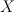$X$ as follows.

A member of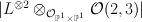$|L^{\otimes 2} \otimes_{\cO_{\PP^1 \times \PP^1}} \cO(2,3)|$ on the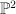$\PP^2$-bundle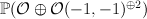$\PP(\cO \oplus \cO(-1,-1)^{\oplus 2})$ over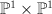$\PP^1 \times \PP^1$ such that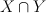$X \cap Y$ is irreducible, where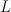$L$ is the tautological line bundle and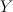$Y$ is a member of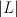$|L|$.

Our first mistake, as Mukai-sensei pointed out in an email to Corti, was using the wrong weight convention for projective bundles.  Mori and Mukai use negative weights, so the ambient$\PP^2$-bundle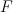$F$ is the toric variety with weight data: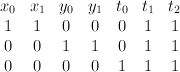$\begin{array}{cccccccc} x_0 & x_1 & y_0 & y_1 & t_0 & t_1 & t_2 & \\ 1 & 1 & 0 & 0 & 0 & 1 & 1 & \\ 0& 0 & 1 & 1 & 0 & 1 & 1 & \\ 0 & 0 & 0 & 0& 1 &1 & 1 & \end{array}$
For later convenience we change basis, expressing$F$ as the toric variety with weight data: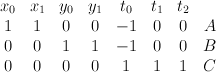$\begin{array}{cccccccc} x_0 & x_1 & y_0 & y_1 & t_0 & t_1 & t_2 & \\ 1 & 1 & 0 & 0 & -1 & 0 & 0 & A \\ 0& 0 & 1 & 1 & -1 & 0 & 0 & B \\ 0 & 0 & 0 & 0& 1 &1 & 1 & C \end{array}$$X$ is a section of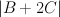$|B+2C|$.

Our second mistake was failing to accurately account for the fact that although$X$ is Fano, the bundle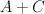$A+C$ (which restricts to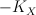$-K_X$ on$X$) is only semi-positive on$F$.  Thus we are in the situation described in this post and, in the notation defined there, we have: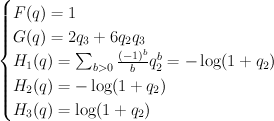$\begin{cases} F(q) = 1 \\ G(q) = 2q_3+6q_2 q_3 \\ H_1(q) = \sum_{b>0} {(-1)^{b} \over b} q_2^b = {-\log(1+q_2)} \\ H_2(q) = {-\log(1+q_2)} \\ H_3(q) = \log(1+q_2) \end{cases}$
and hence: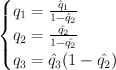$\begin{cases} q_1 = {\hat{q}_1 \over 1 - \hat{q}_2} \\ q_2 = {\hat{q_2} \over 1 - \hat{q_2}} \\ q_3 = \hat{q}_3 (1-\hat{q_2}) \end{cases}$
Thus the cohomological-degree-zero part of the J-function is: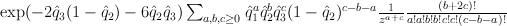$\exp({-2}\hat{q}_3(1-\hat{q}_2)-6\hat{q}_2 \hat{q}_3) \sum_{a,b,c\geq 0} \hat{q}_1^a \hat{q}_2^b \hat{q}_3^c(1-\hat{q}_2)^{c-b-a} {1 \over z^{a+c}} {(b+2c)! \over a!a!b!b!c!c!(c-b-a)!}$
and setting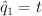$\hat{q}_1 = t$,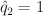$\hat{q}_2 = 1$,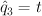$\hat{q}_3 = t$,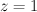$z=1$ yields: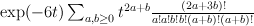$\exp(-6t) \sum_{a,b\geq 0} t^{2a+b} {(2a+3b)! \over a!a!b!b!(a+b)!(a+b)!}$
Regularizing this gives: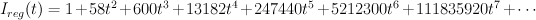$I_{reg}(t) =1+58 t^2+600 t^3+13182 t^4+247440 t^5+5212300 t^6+111835920 t^7+ \cdots$
As Galkin conjectured, this is period sequence 97.

## Expected distribution of equations.

Let X be a smooth Fano threefold with Picard number P =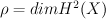$\rho = dim H^2(X)$.

Then subring of algebraic (even) cycles in X is (2+2P)-dimensional, and its Lefschetz decomposition has P blocks: 1 block of length 4 and (P-1) blocks of length 2. So its image in cohomologies of anticanonical section (K3 surface) is (2+2P – P) = (2+P)-dimensional.

For “general” Fano threefold with Picard number P we expect
regularized quantum differential equation (RQDE) to be of degree (2+P) in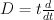$D = t \frac{d}{dt}$
and to have (2+2P) singular points. Nevertheless degree in t may be more than number of singular points
due to apparent singularities.

It turns out that condition for general is not very general in practice.

Assume Fano threefold X has action of finite group G in one of the 4 ways:
a. G acts on X by regular (algebraic) transformations,
b. G acts on X by symplectic transformations,
c. X is defined over non-algebraically closed field k and G is Galois group Gal(k),
d. X is a fiber of a smooth family over some base B and fundamental group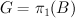$G = \pi_1(B)$ acts on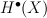$H^\bullet(X)$ via monodromy.

For cases a,b,c consider the induced action of G on cohomology of X.

Let p =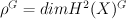$\rho^G = dim H^2(X)^G$ be $G$-invariant Picard number.
G-invariant part of cohomologies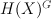$H(X)^G$ is (2+2p)-dimensional.

Define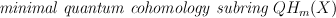$\emph{minimal quantum cohomology subring } QH_m(X)$ of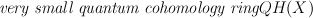$\emph{very small quantum cohomology ring} QH(X)$ as subring generated by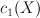$c_1(X)$ and C[t].
It is easy to see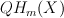$QH_m(X)$ is contained in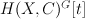$H(X, C)^G [t]$.

This implies that regularized I-series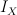$I_X$ is annihilated by
differential operator of degree (2+p).

So it is natural to ask about possible G-actions on Fano threefolds.
First (numerical) step is to see the possible automorphisms of Mori cone or Kaehler cone.
We have some structures on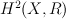$H^2(X,R)$:
a. lattice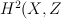$H^2(X,Z$) and element$c_1(X)$ inside the lattice,
b. rational polyhedral cone of numerically effective divisors,
c. nondegenerate integral quadratic form (Lefschetz pairing) :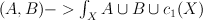$(A,B) -> \int_X A \cup B \cup c_1(X)$.
We call this information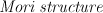$\emph{Mori structure}$.

Group of automorphisms of Mori structure is finite, and for any action
G-invariant Picard number is not less than dimension of invariants of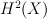$H^2(X)$
with respect to whole group of automorphisms of Mori structure.

As far as I remember (but cannot find a reference) for all Fano threefolds one may find some moduli
and some kind of G-action such that G-invariant Picard group coincides with invariant part of $H^2$ with respect to automorphisms of Mori structure.

The standard reference for automorphisms of Mori structure is probably:
Kenji Matsuki, “Weyl groups and birational transformations among minimal models”, AMS 1995

He studies slightly different problem, but has a similar answer. Unfortunately I haven’t a copy of this book, but copied one page from google books.

He says automorphisms of Mori structures turn out to be Weyl groups.

He claims the following Fano threefolds have nontrivial automorphisms:

P – Picard number, then list of Mori-Mukai numbers with the given Picard number

P=2: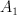$A_1$: 2, 6, 12, 21, 32 (these should be G-Fano, but number 2 is suspicious)
other have p=2

P=3: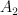$A_2$: 1, 27 (G-Fano, suspicious that 13 is in the next line)$A_1$: 3, 7, 9, 10, 13, 17, 19, 20, 25, 31 (should correspond to p=2)
other have p=3

P=4: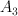$A_3$: 1 (G-Fano)$A_2$: 6 (p=2)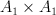$A_1 \times A_1$: 2 (p=2)$A_1$: 3, 4, 7, 8, 10, 12 (p=3)
trivial – 5,9,11 (should have p=4)
missing number 13 from Erratum

P=5: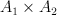$A_1 \times A_2$: 3 (p=2)$A_2$: 1 (p=3)$A_1$: 2 (p=4)

For cases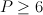$P \geq 6$ our threefolds are products of a line and del Pezzo surface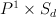$P^1 \times S_d$. They all have Weyl group of type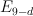$E_{9-d}$ and p=2.

So the distribution in p is the following (case 4.13 is missing):
p is always less than 5;
p=4 – 4 varieties: 5.2; 4.5, 4.9, 4.11
p=3 – 26 varieties: 5.1; 4.3, 4.4, 4.7, 4.8, 4.10, 4.12; and 19 with P=3
p=2 – 50 varieties
p=1 – 25 varieties (or 26 if 2.2 is there)

This means just 4 varieties should have N=6, and other have even less.

The obvious thing to do is to recompute ourselves the Mori structure and its automorphisms
(in particular discriminant of Lefschetz quadratic form is an important invariant that we need anyway).

## D3 forms for the Minkowksi ansatz

As mentioned below, the Minkowksi ansatz gives rise to 23 D3 forms. The connection matrices and various other useful data about these D3 forms are here.  This list contains 15 of the 17 minimal Fano varieties and several other currently-mysterious examples.  Some of these look like they could be G-Fanos.  The original data, without annotations, are here:   MinkowskiD3Matrices.

Sergei: please can you take a look at this list and see whether you can fill in the gaps.

## New data

I ran the Minkowski ansatz for all 4319 3-dimensional reflexive polytopes, calculating the first 100 power series coefficients of the principal period for each of them.  The results were as follows.

3025 of the polytopes had a non-empty list of Minkowski polynomials, and 670 of them had more than one Minkowksi polynomial.

There were 165 distinct sequences of power series coefficients.  I then looked for differential operators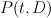$P(t,D)$ annilihating the principal period such that$P(t,D)$ has degree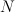$N$ in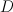$D$ and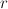$r$ in$t$, where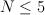$N \leq 5$ and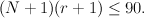$(N+1)(r+1)\leq 90.$  The results were:

N=3:	23 examples in total

r=2:	3 examples
r=3:	2 examples
r=4:	18 examples

N=4:	49 examples in total

r=7:	5 examples
r=8:	3 examples
r=9:	14 examples
r=10:	27 examples

N=5:	10 examples in total

r=11:	1 example
r=12:	2 examples
r=13:	0 examples
r=14:	7 examples

So in particular we found 23 D3 forms.